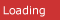# C# CornerRelated resources for Python Libraries
• Multiple Linear Regression11/11/2019 3:43:29 AM. This is the fifteenth article in the series. Multiple linear regression (MLR), also known simply as multiple regression, is a statistical technique that uses several explanatory variables to predict t
• Logistic Regression11/10/2019 8:51:05 AM. This is the fourteenth tutorial in the series. Logistic regression is a statistical model that in its basic form uses a logistic function to model a binary dependent variable, although many more compl
• Linear Regression10/30/2019 2:32:26 AM. This is the thirteenth tutorial in the series. In statistics, linear regression is a linear approach to modeling the relationship between a scalar response and one or more explanatory variables. The c
• Data Science- A Beginner's Tutorial10/28/2019 5:55:48 AM. This is the twelfth tutorial in this series. Data science is a multi-disciplinary field that uses scientific methods, processes, algorithms, and systems to extract knowledge and insights from structur
• All about Deep Learning Tutorial10/23/2019 4:33:04 AM. This is the eleventh tutorial in the series. In this tutorial, we will be studying Deep Learning. Deep learning is a subset of machine learning in artificial intelligence (AI) that has networks capabl
• All about Machine Learning10/22/2019 1:03:23 AM. This is the tenth tutorial in the series. In this tutorial, we will be studying Machine Learning. Machine learning is the scientific study of algorithms and statistical models that computer systems us
• Artificial Intelligence - a Beginner's Tutorial10/21/2019 4:43:34 AM. This is the ninth tutorial in the series. In this tutorial, we will be studying Artificial Intelligence and its functionalities. Artificial intelligence (AI) is the simulation of human intelligence pr
• A Complete Python Seaborn Tutorial10/17/2019 5:21:44 AM. This is the seventh tutorial in the series. In this tutorial, we will be studying about seaborn and its functionalities. Seaborn is a Python data visualization library based on matplotlib. It provides
• A Complete Python TensorFlow Tutorial10/17/2019 5:20:16 AM. This is the eighth tutorial in the series. In this tutorial, we will be studying about Tensorflow and its functionalities. TensorFlow is a free and open-source software library for dataflow and differ
• A Complete Python MatPlotLib Tutorial10/11/2019 11:40:11 AM. This is the sixth tutorial in the series. In this tutorial, we will be studying about matplotlib and its functionalities.
• A Complete Python Pandas Tutorial10/11/2019 11:28:40 AM. This is the fourth tutorial in the series. In this tutorial, we will be studying about pandas and its functionalities.
• Python Libraries Needed for Machine Learning10/11/2019 10:55:26 AM. This is the second tutorial in the series. In this tutorial, we will be studying various python libraries and their corresponding functions that we will be using during our series
• A Complete Python Scikit Learn Tutorial9/24/2019 1:40:13 AM. This is the fifth tutorial in the series. In this tutorial, we will be studying about scikit-learn and its functionalities.
• A Complete Python Numpy Tutorial9/8/2019 6:19:09 AM. This is the third tutorial in the series. In this tutorial, we will learn about numpy or numerical python.